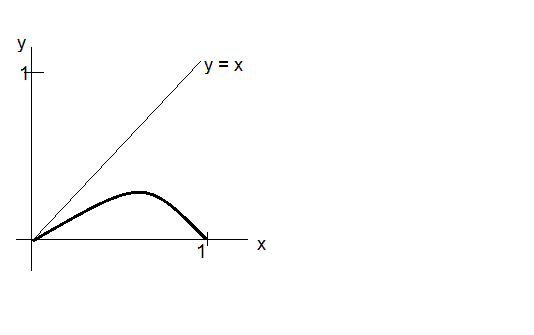# Rudin POMA: chapter 4 problem 14

anon3335

## Homework Statement

Question: Let ##I = [0,1]##. Suppose ##f## is a continuous mapping of ##I## into ##I##. Prove that ##f(x) = x## for at least one ##x∈I##.

## Homework Equations

Define first(##[A,B]##) = ##A## and second(##[A,B]##) = ##B## where ##[A,B]## is an interval in ##R##.

## The Attempt at a Solution

Proof: let ##M = sup\ f(I)## and ##m = inf\ f(I)##. Assume that ##M ≠ m## and that ##f(x) ≠ x## for all ##x∈ I##.Then ##f## is strictly monotonic and so let ##L_1## = ##[f(m),f(M)]## and define ##L_n ##=## [f(first(L_{n-1}), f(second(L_{n-1}))]## (##n ≥ 2##). Then V=## \bigcap L_n## is non empty. Now let ##y∈V##. Then there exists ##x_1∈ V## such that ##f(x_1) = y##. Then if ##x_1 ≠y##, we have ##f(x_1) ≠ y##, which is a contradiction and so ##x_1 = y##. But this is contradicting our assumption. For the case where ##M = m## it is trivial as the function would be a constant one.

Homework Helper

## Homework Statement

Proof: let ##M = sup\ f(I)## and ##m = inf\ f(I)##. Assume that ##M ≠ m## and that ##f(x) ≠ x## for all ##x∈ I##.Then ##f## is strictly monotonic
I don't see how ##M>m## and ##f(x) ≠ x## imply that f(x) is monotonic.

anon3335
I don't see how ##M>m## and ##f(x) ≠ x## imply that f(x) is monotonic.
Since then ##f## would be a continuous injective function on ##I## and so it is strictly monotonic.

Homework Helper
Since then ##f## would be a continuous injective function on ##I## and so it is strictly monotonic.
I agree that a continuous injective function is monotonic, but why is the function injective? How does ##f(x) \neq x## imply this?

anon3335
I agree that a continuous injective function is monotonic, but why is the function injective? How does ##f(x) \neq x## imply this?
Let ##x_1## ≠ ##x_2##. Then one gets ##f(x_1) ≠ f(x_2)## according to our assumption and so ##f## is injective.

Homework Helper
Let ##x_1## ≠ ##x_2##. Then one gets ##f(x_1) ≠ f(x_2)## according to our assumption and so ##f## is injective.
Your argument is ##x_1 \neq f(x_1)## and ##x_2 \neq f(x_2)## implies ##f(x_1) \neq f(x_2)##?

anon3335
Your argument is ##x_1 \neq f(x_1)## and ##x_2 \neq f(x_2)## implies ##f(x_1) \neq f(x_2)##?
Yup.

Homework Helper
Yup.
So ##f(x_1) \neq x_1 \neq x_2 \neq f(x_2)## implies ##f(x_1) \neq f(x_2)##? But ##\neq## is not transitive

Last edited by a moderator:
Mentor
Question: Let ##I = [0,1]##. Suppose ##f## is a continuous mapping of ##I## into ##I##. Prove that ##f(x) = x## for at least one ##x∈I##.
Basically, you're trying to show that every function f that maps the interval [0, 1] into itself must cross through or touch the line y = x at least once. I agree with @tnich that you are asserting that f is injective without showing why this must be true. In fact, for the given conditions, it doesn't have to be true (that f is injective).

In the graph below, f (dark curve) maps [0, 1] into [0, 1]. f is continuous on [0, 1], but neither injective nor surjective.#### Attachments

Homework Helper
What could you do with the function ##g(x) \equiv f(x) - x##?

anon3335
So ##f(x_1) \neq x_1 \neq x_2 \neq f(x_2)## implies ##f(x_1) \neq f(x_2)##? But ##\neq## is not transitive
Yes you are right. My argument is flawed in that step, but what if we delete that part from the proof? wouldn't the proof still hold?

Homework Helper
Yes you are right. My argument is flawed in that step, but what if we delete that part from the proof? wouldn't the proof still hold?
Can you make your sequence of intervals converge without it? Could you make your sequence converge even with it? Consider the case ##f(x) = 1-x##.

anon3335
Can you make your sequence of intervals converge without it? Could you make your sequence converge even with it? Consider the case ##f(x) = 1-x##.
Ah I see. Yes the argument would not hold. Thanks for your help.

anon3335
What could you do with the function ##g(x) \equiv f(x) - x##?
I have solved the problem with this specific way, but I wanted to solve the problem with another approach.